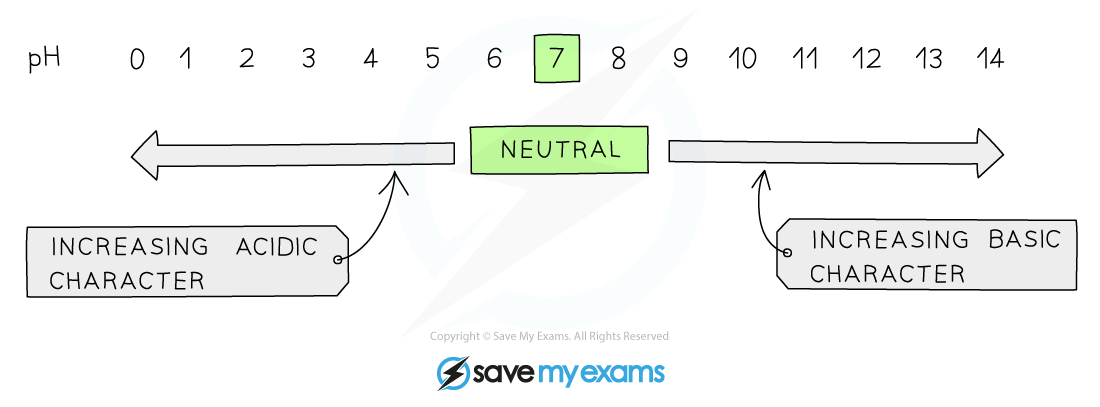# IB DP Chemistry: SL复习笔记8.2.3 Interpreting pH

### Interpreting pH

• The pH scale is a numerical scale that shows how acidic or alkaline a solution is
• The values on the pH scale go from 0-14 (extremely acidic substances have values of below 0)
• All acids have pH values of below 7, all alkalis have pH values above 7
• The lower the pH then the more acidic the solution is
• The higher the pH then the more alkaline the solution isThe pH scale showing acidity, neutrality and alkalinity

#### pH of acids

• Acidic solutions (strong or weak) always have more H+ than OH- ions
• Since the concentration of H+ is always greater than the concentration of OH- ions, [H+] is always greater than 10-7 mol dm-3
• Using the pH formula, this means that the pH of acidic solutions is always below 7
• The higher the [H+] of the acid, the lower the pH

#### pH of bases

• Basic solutions (strong or weak) always have more OH- than H+ ions
• Since the concentration of OH- is always greater than the concentration of H+ ions, [H+] is always smaller than 10-7 mol dm-3
• Using the pH formula, this means that the pH of basic solutions is always above 7
• The higher the [OH-] of the base, the higher the pH

#### The pH of water

• Water at 298K has equal amounts of OH- and H+ ions with concentrations of 10-7 mol dm-3
• To calculate the pH of water, the following formula should be used:pH= -log (10-7)= 7

• Thus, water has a pH of 7 at 298 K